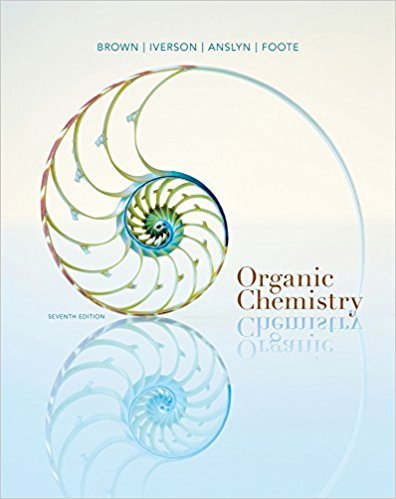×

×

# Solutions for Chapter 22: Reactions of Benzene and Its Derivatives## Full solutions for Organic Chemistry | 7th Edition

ISBN: 9781133952848Solutions for Chapter 22: Reactions of Benzene and Its Derivatives

Solutions for Chapter 22
4 5 0 290 Reviews
17
3
##### ISBN: 9781133952848

Chapter 22: Reactions of Benzene and Its Derivatives includes 57 full step-by-step solutions. Organic Chemistry was written by and is associated to the ISBN: 9781133952848. This textbook survival guide was created for the textbook: Organic Chemistry, edition: 7. Since 57 problems in chapter 22: Reactions of Benzene and Its Derivatives have been answered, more than 56558 students have viewed full step-by-step solutions from this chapter. This expansive textbook survival guide covers the following chapters and their solutions.

Key Chemistry Terms and definitions covered in this textbook
• acid rain

Rainwater that has become excessively acidic because of absorption of pollutant oxides, notably SO3, produced by human activities. (Section 18.2)

• acid-dissociation constant (Ka)

An equilibrium constant that expresses the extent to which an acid transfers a proton to solvent water. (Section 16.6)

• Alkoxy group

An !OR group where R is an alkyl group

• alpha particles

Particles that are identical to helium-4 nuclei, consisting of two protons and two neutrons, symbol 4 2He or 4 2a. (Section 21.1)

• androgens

Male sex hormones.

• aqueous solution

A solution in which water is the solvent. (Chapter 4: Introduction)

• chair conformation

The lowest energy conformation for cyclohexane, in which all bond angles are fairly close to 109.5° and all hydrogen atoms are staggered.

• copolymer

A polymer that is constructed from more than one repeating unit.

• Coulomb’s law.

The potential energy between two ions is directly proportional to the product of their charges and inversely proportional to the distance between them. (9.3)

• critical mass.

The minimum mass of fissionable material required to generate a self-sustaining nuclear chain reaction. (19.5)

• dihedral angle

The angle by which two groups are separated in a Newman projection.

A substance with one or more unpaired electrons. (Section 21.9)

• limiting reactant (limiting reagent)

The reactant present in the smallest stoichiometric quantity in a mixture of reactants; the amount of product that can form is limited by the complete consumption of the limiting reactant. (Section 3.7)

• Merrifield synthesis

A method for building a peptide from protected building blocks.

• organic chemistry

The study of carbon-containing compounds, typically containing carbon–carbon bonds. (Section 2.9; Chapter 24:Introduction)

• physiological pH

The pH of blood (approximately 7.3).

A compound such as a phenol that selectively reacts with radicals to remove them from a chain reaction and terminate the chain

• substitution reactions

Reactions in which one group is replaced by another group.

• Sulfi de

The sulfur analog of an ether; a molecule containing a sulfur atom bonded to two carbon atoms. Sulfi des are also called thioethers

• Wave function

A solution to a set of equations that defi nes the energy of an electron in an atom and the region of space it may occupy.